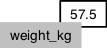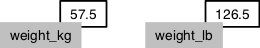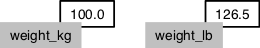# Starting out in R

R is both a programming language and an interactive environment for statistics. Today we will be concentrating on R as an interactive environment.

Working with R is primarily text-based. The basic mode of use for R is that the user types in a command in the R language and presses enter, and then R computes and displays the result.

We will be working in RStudio. This surrounds the console, where one enters commands and views the results, with various conveniences. In addition to the console, RStudio provides panels containing:

• A text editor, where R commands can be recorded for future reference.
• A history of commands that have been typed on the console.
• An “environment” pane with a list of variables, which contain values that R has been told to save from previous commands.
• A file manager.
• Help on the functions available in R.
• A panel to show plots (graphs).

Open RStudio, click on the “Console” pane, type `1+1` and press enter. R displays the result of the calculation. In this document, we will be showing such an interaction with R as below.

``1+1``
``##  2``

`+` is called an operator. R has the operators you would expect for for basic mathematics: `+` `-` `*` `/` `^`. It also has operators that do more obscure things.

`*` has higher precedence than `+`. We can use brackets if necessary `( )`. Try `1+2*3` and `(1+2)*3`.

Spaces can be used to make code easier to read.

We can compare with `== < > <= >=`. This produces a “logical” value, `TRUE` or `FALSE`. Note the double equals, `==`, for equality comparison.

``2 * 2 == 4``
``##  TRUE``

There are also character strings such as `"string"`.

## Variables

A variable is a name for a value, such as `x`, `current_temperature`, or `subject.id`. We can create a new variable by assigning a value to it using `<-`.

``weight_kg <- 55``

RStudio helpfully shows us the variable in the “Environment” pane. We can also print it by typing the name of the variable and hitting enter. In general, R will print to the console any object returned by a function or operation unless we assign it to a variable.

``weight_kg``
``##  55``

Examples of valid variables names: `hello`, `hello_there`, `hello.there`, `value1`. Spaces aren’t ok inside variable names. Dots (`.`) are ok, unlike in many other languages.

We can do arithmetic with the variable:

``````# weight in pounds:
2.2 * weight_kg``````
``##  121``

We can also change a variable’s value by assigning it a new value:

``````weight_kg <- 57.5
# weight in kilograms is now
weight_kg``````
``##  57.5``

If we imagine the variable as a sticky note with a name written on it, assignment is like putting the sticky note on a particular value:Assigning a new value to one variable does not change the values of other variables. For example, let’s store the subject’s weight in pounds in a variable:

``````weight_lb <- 2.2 * weight_kg
# weight in kg...
weight_kg``````
``##  57.5``
``````# ...and in pounds
weight_lb``````
``##  126.5``and then change `weight_kg`:

``````weight_kg <- 100.0
# weight in kg now...
weight_kg``````
``##  100``
``````# ...and weight in pounds still
weight_lb``````
``##  126.5``Since `weight_lb` doesn’t “remember” where its value came from, it isn’t automatically updated when `weight_kg` changes. This is different from how spreadsheets work.

## Vectors

A vector1 of numbers is a collection of numbers. We call the individual numbers elements of the vector.

We can make vectors with `c( )`, for example `c(1,2,3)`. `c` means “combine”. R is obsesssed with vectors. In R, numbers are just vectors of length one. Many things that can be done with a single number can also be done with a vector. For example arithmetic can be done on vectors just like on single numbers.

``````myvec <- c(10,20,30,40,50)
myvec + 1``````
``##  11 21 31 41 51``
``myvec + myvec``
``##   20  40  60  80 100``
``length(myvec)``
``##  5``
``c(60, myvec)``
``##  60 10 20 30 40 50``
``c(myvec, myvec)``
``##   10 20 30 40 50 10 20 30 40 50``

When we talk about the length of a vector, we are talking about the number of numbers in the vector.

#### Types of vector

We will also encounter vectors of character strings, for example `"hello"` or `c("hello","world")`. Also we will encounter “logical” vectors, which contain `TRUE` and `FALSE` values. R also has “factors”, which are categorical vectors, and behave very much like character vectors (think the factors in an experiment).

### Challenge

Sometimes the best way to understand R is to try some examples and see what it does.

What happens when you try to make a vector containing different types, using `c( )`? Make a vector with some numbers, and some words (eg. character strings like “test”, or “hello”).

Why does the output show the numbers surrounded by quotes " " like character strings are?

Because vectors can only contain one type of thing, R chooses a lowest common denominator type of vector, a type that can contain everything we are trying to put in it. A different language might stop with an error, but R tries to soldier on as best it can. A number can be represented as a character string, but a character string can not be represented as a number, so when we try to put both in the same vector R converts everything to a character string.

#### Indexing vectors

Access elements of a vector with `[ ]`, for example `myvec` to get the first element. You can also assign to a specific element of a vector.

``myvec``
``##  10``
``myvec``
``##  20``
``````myvec <- 5
myvec``````
``##  10  5 30 40 50``

Can we use a vector to index another vector? Yes!

``````myind <- c(4,3,2)
myvec[myind]``````
``##  40 30  5``

We could equivalently have written

``myvec[c(4,3,2)]``
``##  40 30  5``

Sometimes we want a contiguous slice from a vector.

``myvec[3:5]``
``##  30 40 50``

`:` here actually creates a vector, which is then used to index `myvec`. `:` is pretty useful on its own too.

``3:5``
``##  3 4 5``
``1:50``
``````##    1  2  3  4  5  6  7  8  9 10 11 12 13 14 15 16 17 18 19 20 21 22 23
##  24 25 26 27 28 29 30 31 32 33 34 35 36 37 38 39 40 41 42 43 44 45 46
##  47 48 49 50``````
``````numbers <- 1:10
numbers*numbers``````
``##     1   4   9  16  25  36  49  64  81 100``

Now we can see why R always puts a `` in its output: it is indicating that the first element of the vector can be accessed with ``. Subsequent lines show the appropriate index to access the first number in the line.

### Challenge - Indexing and slicing data

We can take slices of character vectors as well:

``````phrase <- c("I", "don't", "know", "I", "know")
# first three words
phrase[1:3]``````
``##  "I"     "don't" "know"``
``````# last three words
phrase[3:5]``````
``##  "know" "I"    "know"``
1. If the first four words are selected using the slice `phrase[1:4]`, how can we obtain the first four words in reverse order?

2. What is `phrase[-2]`? What is `phrase[-5]`? Given those answers, explain what `phrase[-1:-3]` does.

3. Use indexing of `phrase` to create a new character vector that forms the phrase “I know I don’t”, i.e. `c("I", "know", "I", "don't")`.

## Functions

R has various functions, such as `sum( )`. We can get help on a `sum` with `?sum`.

``?sum``
``sum(myvec)``
``##  135``

Here we have called the function `sum` with the argument `myvec`.

Because R is a language for statistics, it has many built in statistics-related functions. We will also be loading more specialized functions from “libraries” (also known as “packages”).

Some functions take more than one argument. Let’s look at the function `rep`, which means “repeat”, and which can take a variety of different arguments. In the simplest case, it takes a value and the number of times to repeat that value.

``rep(42, 10)``
``##   42 42 42 42 42 42 42 42 42 42``

As with many functions in R—which is obsessed with vectors—the thing to be repeated can be a vector with multiple elements.

``rep(c(1,2,3), 10)``
``##   1 2 3 1 2 3 1 2 3 1 2 3 1 2 3 1 2 3 1 2 3 1 2 3 1 2 3 1 2 3``

So far we have used positional arguments, where R determines which argument is which by the order in which they are given. We can also give arguments by name. For example, the above is equivalent to

``rep(c(1,2,3), times=10)``
``##   1 2 3 1 2 3 1 2 3 1 2 3 1 2 3 1 2 3 1 2 3 1 2 3 1 2 3 1 2 3``
``rep(x=c(1,2,3), 10)``
``##   1 2 3 1 2 3 1 2 3 1 2 3 1 2 3 1 2 3 1 2 3 1 2 3 1 2 3 1 2 3``
``rep(x=c(1,2,3), times=10)``
``##   1 2 3 1 2 3 1 2 3 1 2 3 1 2 3 1 2 3 1 2 3 1 2 3 1 2 3 1 2 3``

Arguments can have default values, and a function may have many different possible arguments that make it do obscure things. For example, `rep` can also take an argument `each=`. It’s typical for a function to be invoked with some number of positional arguments, which are always given, plus some less commonly used arguments, typically given by name.

``rep(c(1,2,3), each=3)``
``##  1 1 1 2 2 2 3 3 3``
``rep(c(1,2,3), each=3, times=5)``
``````##   1 1 1 2 2 2 3 3 3 1 1 1 2 2 2 3 3 3 1 1 1 2 2 2 3 3 3 1 1 1 2 2 2 3 3
##  3 1 1 1 2 2 2 3 3 3``````

### Challenge - Using functions

1. Use `sum` to sum from 1 to 5 (ie 1+2+3+4+5).

2. Use `sum` to sum from 1 to 10,000.

3. You are reading some R code and see that it uses a function called `seq`. What does `seq` do?

## Lists

Vectors contain all the same kind of thing. Lists can contain different kinds of thing. Lists can even contain vectors or other lists as elements.

We generally give the things in a list names. Try `list(num=42, greeting="hello")`. To access named elements we use `\$`.

``````mylist <- list(num=42, greeting="Hello, world")
mylist\$greeting``````
``##  "Hello, world"``

A final piece of special syntax for lists is `[[ ]]` to access individual elements by number. We won’t be using this today, but include it for completeness.

``mylist[]``
``##  "Hello, world"``

Functions that need to return multiple outputs often do so as a list.

## Appendix: Overview of data types

We’ve seen several data types in this chapter, and will be seeing two more in the following chapters. This section serves as an overview of data types in R and their typical usage.

Each data type has various ways it can be created and various ways it can be accessed. If we have data in the wrong type, there are functions to “cast” it to the right type.

This will all make more sense once you have seen these data types in action.

### vector

Vectors contain zero or more elements, all of the same basic type.

Elements can be named (`names`), but often aren’t.

Access single elements: `vec`

Take a subset of a vector: `vec[c(1,3,5)]` `vec[c(TRUE,FALSE,TRUE,FALSE,TRUE)]`

Vectors come in several different flavours.

#### numeric vector

Numbers. Internally stored as “floating point” so there is a limit to the number of digits accuracy, but this is usually entirely adequate.

Examples: `42` `1e-3` `c(1,2,0.7)`

Casting: `as.numeric("42")`

#### character vector

Character strings.

Examples: `"hello"` `c("Let","the","computer","do","the","work")`

Casting: `as.character(42)`

#### logical vector

TRUE or FALSE values.

Examples: `TRUE` `FALSE` `T` `F` `c(TRUE,FALSE,TRUE)`

#### factor vector

A categorical vector, where the elements can be one of several different “levels”. There will be more on these in the chapter on data frames.

Creation/casting: `factor(c("mutant","wildtype","mutant"), levels=c("wildtype","mutant"))`

### list

Lists contain zero or more elements, of any type. Elements of a list can even be vectors with their own multiple elements, or other lists. If your data can’t be bundled up in any other type, bundle it up in a list.

List elements can and typically do have names (`names`).

Access an element: `mylist[]` `mylist[["elementname"]]` `mylist\$elementname`

Creation: `list(a=1, b="two", c=FALSE)`

### matrix

A matrix is a two dimensional tabular data structure in which all the elements are the same type. We will typically be dealing with numeric matrices, but it is also possible to have character or logical matrices, etc.

Matrix rows and columns may have names (`rownames`, `colnames`).

Access an element: `mat[3,5]` `mat["arowname","acolumnname"]`

Get a whole row: `mat[3,]`

Get a whole column: `mat[,5]`

Creation: `matrix( )`

Casting: `as.matrix( )`

### data.frame

A data frame is a two dimensional tabular data structure in which the columns may have different types, but all the elements in each column must have the same type.

Data frame rows and columns may have names (`rownames`, `colnames`). However in typical usage columns are named but rows are not.2

Accessing elements, rows, and columns is the same as for matrices, but we can also get a whole column using `\$`.

Creation: `data.frame(colname1=values1,colname2=values2,...)`

Casting: `as.data.frame( )`

1. We use the word vector here in the mathematical sense, as used in linear algebra, not in any biological sense, and not in the geometric sense.

2. For some reason, data frames use partial matching on row names, which can cause some very puzzling bugs.